Select Page

### Probability is one of the trickiest (and equally fascinating) topics in the CAT quantitative aptitude section. This topic can feature tricky problems that test a combination of your mathematical and reasoning skills. To help you with this topic, we feature this set of 5 probability questions.

Question 1:  One ticket is selected at random from 50 tickets numbered  0, 01, 02, ……, 49.   Then,   the probability that the sum of the digits on the selected ticket is 8, given that the product of these digits is zero equals

(a) 1/14
(b) 1/7
(c) 5/14
(d) 1/50

Explanation: (a)

Here we have

Let A be the event that sum of the digits on the selected ticket is 8, then

Let B be the event that the product of the digits is zero.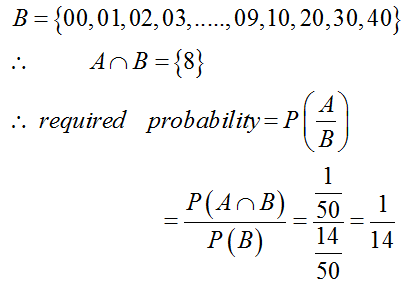Question 2: It is given that the events A and B are such that P(A) = ¼ , P(A|B) = ½ and P(B|A) = 2/3. Then P(B|A) = 2/3. Then P(B) is
(a) 1/2
(b) 1/6
(c) 1/3
(d) 2/3

Explanation: (c)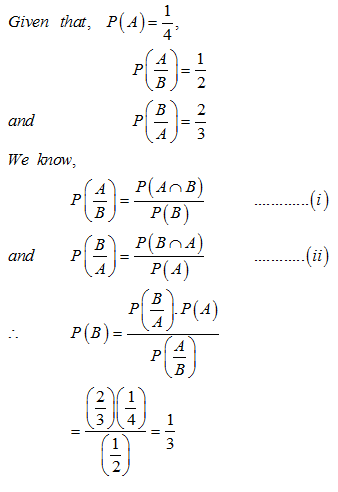Question 3: A die is thrown. Let A be the event that the number obtained is greater than 3. Let B be the event that the number obtained is less than 5. Then P (AUB) is
(a) 2/5
(b) 3/5
(c) 0
(d) 1

Explanation: (d)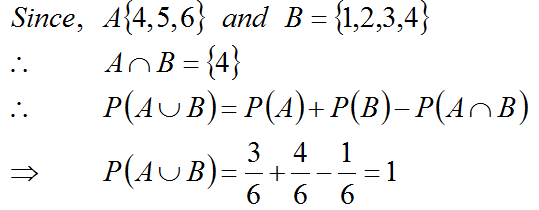Question 4: A pair of fair dice is thrown independently three times. The probability of getting a total of exactly 9 twice is

(a) 1/729
(b) 8/9
(c) 8/729
(d) 8/243

Explanation: (d)

Probability of getting a total of 9 in a single throw

4/36 = 1/9

Probability of getting a total of 9 exactly in double throw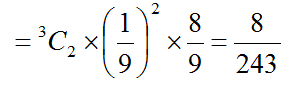Question 5: Two aeroplanes I and II bomb a target in succession. The probabilities of I and II scoring a hit correctly are 0.3 and 0.2, respectively. The second plane will bomb only if the first misses the target. The probability that the target is hit by the second plane, is
(a) 0.06
(b) 0.14
(c) 0.32
(d) 0.7

Explanation: (c)

Let the events,

A = 1st aeroplane hit the target

B =2nd aeroplane hit the target

And their corresponding probabilities are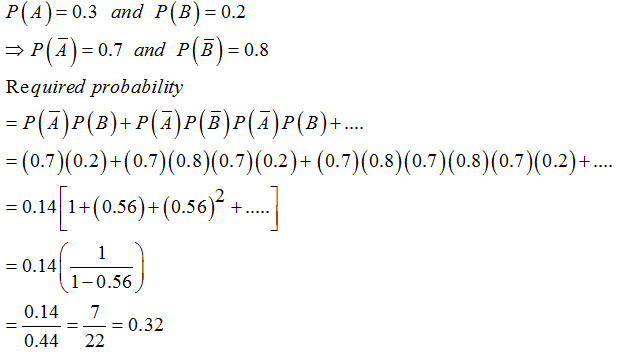### Extra tips for CAT Probability

• Probability is one topic that requires a combination of skills. Pure mathematical knowledge for the topic is not enough.
• Make sure you learn more about problems based on dices, playing cards, and more. These topics frequently feature for this topic.
• Class 11/12 books can be a good resource for this topic. You can pick books by reputed authors in order to master this topic.
• Also, make sure you are clear with Permutation and Combinations. You will need some P and C concepts in this topic.
Get Posts Like This Sent to your Email
Updates for Free Live sessions and offers are sent on mail. Don't worry: we do not send too many emails..:)
Get Posts Like This Sent to your Email
Updates for Free Live sessions and offers are sent on mail. Don't worry: we do not send too many emails..:)